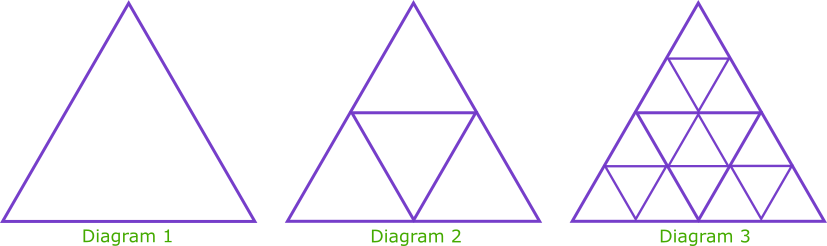# Exam-Style Question on Sequences Exponential Models

## A mathematics exam-style question with a worked solution that can be revealed gradually

##### List Of QuestionsExam-Style QuestionMore Sequences QuestionsMore on this Topic

Question id: 121. This question is similar to one that appeared on a IGCSE Extended paper in 2014. The use of a calculator is allowed.The diagrams above show a growing fractal of triangles. The sides of the largest equilateral triangle in each diagram are of length 1 metre.

In the second diagram there are four triangles each with sides of length $$\frac{1}{2}$$ metre.

In the third diagram there are 16 triangles each with sides of length $$\frac{1}{4}$$ metre.

(a) Complete this table for more diagrams.

 Diagram 1 Diagram 2 Diagram 3 Diagram 4 Diagram 5 Diagram 6 Diagram $$n$$ Length of Side 1 $$\frac{1}{2}$$ $$\frac{1}{4}$$ Power of 2 20 2-1 2-2

(b) Complete this table for the number of the smallest triangles in diagrams 4, 5 and 6.

 Diagram 1 Diagram 2 Diagram 3 Diagram 4 Diagram 5 Diagram 6 Diagram $$n$$ Number of smallest triangles 1 4 16 Power of 2 20 22 24

(c) Calculate the number of the smallest triangles in the diagram where the smallest triangles have sides of length $$\frac{1}{256}$$ metre.

 The worked solutions to these exam-style questions are only available to those who have a Transum Subscription. Subscribers can drag down the panel to reveal the solution line by line. This is a very helpful strategy for the student who does not know how to do the question but given a clue, a peep at the beginnings of a method, they may be able to make progress themselves. This could be a great resource for a teacher using a projector or for a parent helping their child work through the solution to this question. The worked solutions also contain screen shots (where needed) of the step by step calculator procedures. A subscription also opens up the answers to all of the other online exercises, puzzles and lesson starters on Transum Mathematics and provides an ad-free browsing experience.Drag this panel down to reveal the solution

The exam-style questions appearing on this site are based on those set in previous examinations (or sample assessment papers for future examinations) by the major examination boards. The wording, diagrams and figures used in these questions have been changed from the originals so that students can have fresh, relevant problem solving practice even if they have previously worked through the related exam paper.

The solutions to the questions on this website are only available to those who have a Transum Subscription.

Exam-Style Questions Main Page

Search for exam-style questions containing a particular word or phrase:

To search the entire Transum website use the search box in the grey area below.

## Comments:For All:

©1997-2021 WWW.TRANSUM.ORG

©1997 - 2021 Transum Mathematics :: For more exam-style questions and worked solutions go to Transum.org/Maths/Exam/